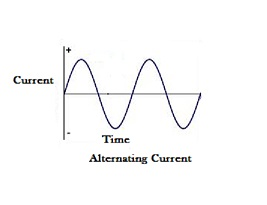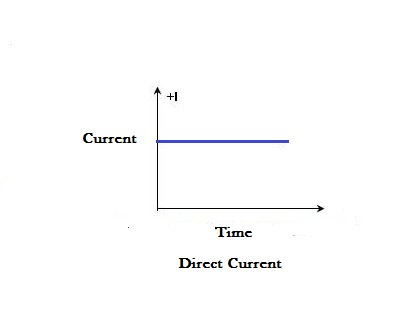We use alternating current and direct current, in our daily life through various machines and devices but we fail to distinguish between them and their applications more often. Let us see some:

1. Alternating Current or A.C is actually the flow of electric charge, which reverses its direction after a certain period of time, whereas Direct Current  or D.C is flow of electric charge only in one direction.

Electric charge is the physical property of a matter which causes it to experience an exertion when kept near other electrically charged matter. Flow of electric charge constitutes current. 1 Ampere of current is produced when 1 Coulomb of charge passes through a point in one second. In case of AC, the electric charge changes its direction periodically because of periodically varying magnetic fields used during its production. whereas in case of DC, the direction of electric charge is unidirectional because of constant magnetic fields used during its production.

2.  The frequency of alternating current is 50 Hz or 60 Hz depending upon your country, most of them use 50 Hz, whereas frequency of Direct Current is 0 Hz.

Frequency of current is defined as the number of complete cycles of periodically varying current from zero to one peak to zero to another peak to zero in one second. Frequency is the reciprocal of time period of one complete sinusoidal wave, In case of DC, the current doesn’t changes direction hence there are no cycles. The frequency is measured in Hertz.

3. The wave type of Alternating current is sinusoidal, triangular or square whereas the Direct current have a pure straight line/quivering wave with respect to time,

A.C has periodically changing electric charges which gives rise to sinusoidal, triangular or square wave which are cyclic in nature whereas D.C has unidirectional flow of electric charge which gives rise to a horizontal quivering wave.Alternating CurrentDirect Current

4. The magnitude of instantaneous alternating current varies constantly with time, whilst the magnitude of instantaneous direct current remains constant.

The amount of current measured at a specific moment of time is known as instantaneous current. Alternating Current has a sinusoidal, Triangular or square waveform and its current changes continually due to periodic change in charge flow. Hence, providing us with varied magnitude every time we check. Direct Current has a Straight Line/Quivering Waveform. It has a unidirectional charge flow therefore it shows constant instantaneous magnitude.

5. A.C Voltages can be transferred to longer distances as compared to D.C voltages via transmission lines.

To keep the wires in transmission lines as thin as practical, the voltage is increased with transformers. Raising the voltage reduces the current(Power load is constant) and therefore reduces the heat build up in the transmission line. AC can be increased and reduced with transformers quite easily whereas DC cannot as transformers work on a constantly changing voltage.

6. Alternating Currents are generated with the help of Alternators whereas Direct currents are generated using dynamos, cells or battery.

Alternator is a machine devised by Micheal Faraday which converts mechanical energy into alternating current. When the magnetic field around a loop of wires changes, a current is induced. A rotating magnet  turns within a stationary set of wires(conductors) wound in coils on an iron core. The field cuts across the conductors, generating an induced electromotive force, as the mechanical force like wind/flowing water turns the magnet. In a magnetic field, when a loop of wire rotates, the electric potential generated in it changes it’s direction with each half turn, generating an alternating current. Dynamo also works on the same principle. The only difference is that it uses a commutator, i.e a rotary switch to generate D.C. This rotary switch prevents this reversal causing the production of AC hence producing D.C.

In the end, I would like to conclude by saying this brief distinction  between alternating and direct current comes in handy a lot of times because it is just like basic general knowledge which everyone must know. So next time you use a device or machine, you will certainly know about the real source behind it.

Share.